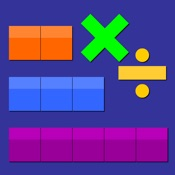# Thinking Blocks Multiplication - Lumos Educational App Store41
Price -Free
\$0

#### DESCRIPTION:

Thinking Blocks Multiplication teaches children how to model and solve word problems involving multiplication and division. In this interactive tutorial, children are introduced to 6 problem solving models. The models help children organize information and visualize number relationships. While Thinking Blocks Multiplication may be used with any elementary math program, it is ideal for children who are learning model drawing strategies found in math books from Singapore. Thinking Blocks Multiplication is best suited for children, ages 7 to 10.

#### OVERVIEW:

Thinking Blocks Multiplication is a free educational mobile app By Math Playground.It helps students in grades 3,4,5 practice the following standards 3.OA.7,4.NBT.5,5.NBT.2.

This page not only allows students and teachers download Thinking Blocks Multiplication but also find engaging Sample Questions, Videos, Pins, Worksheets, Books related to the following topics.

1. 3.OA.7 : Fluently multiply and divide within 100, using strategies such as the relationship between multiplication and division (e.g., knowing that 8 .

2. 4.NBT.5 : Multiply a whole number of up to four digits by a one-digit whole number, and multiply two two-digit numbers, using strategies based on place value and the properties of operations. Illustrate and explain the calculation by using equations, rectangular arrays, and/or area models. (Grade 4 expectations in this domain are limited to whole numbers less than or equal to 1,000,000. A range of algorithms may be used.).

3. 5.NBT.2 : Explain patterns in the number of zeros of the product when multiplying a number by powers of 10, and explain patterns in the placement of the decimal point when a decimal is multiplied or divided by a power of 10. Use whole number exponents to denote powers of 10..

3
4
5

#### STANDARDS:

3.OA.7
4.NBT.5
5.NBT.2

Developer: Math Playground

Software Version: 1.2

Category: Education

### RELATED APPSEdSearch WebSearch